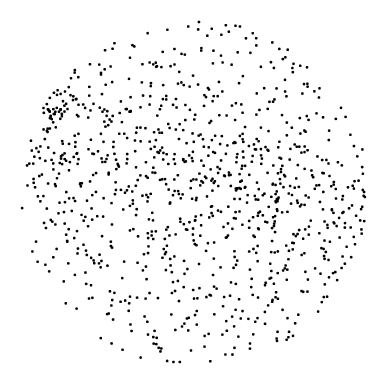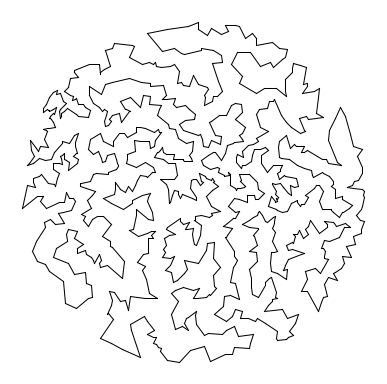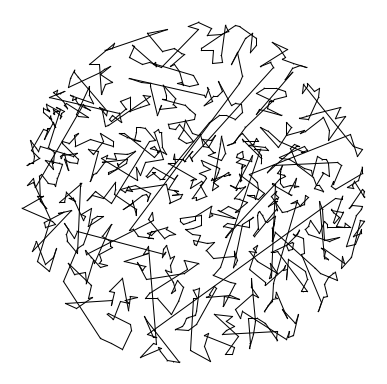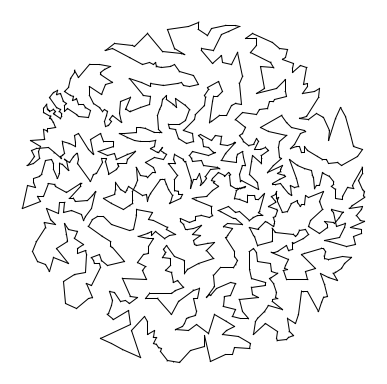COS 126 Traveling Salesperson Problem Programming Assignment

Given a set of N points in the plane, the goal of a traveling salesperson is to visit all of them (and arrive back home) while keeping the total distance traveled as short as possible. Write a program to compute an approximate solution to the traveling salesperson problem (TSP), and use it to find the shortest tour that you can, connecting a given set of points in the plane.Perspective. The importance of the TSP does not arise from an overwhelming demand of salespeople to minimize their travel distance, but rather from a wealth of other applications, many of which seem to have nothing to do with the TSP at first glance. Real world application areas include: vehicle routing, circuit board drilling, VLSI design, robot control, X-ray crystallography, machine scheduling, and computational biology.

Greedy heuristics. The traveling salesperson problem is a notoriously difficult combinatorial optimization problem, In principle, one can enumerate all possible tours, but, in practice, the number of tours is so staggeringly large (roughly N factorial) that this approach is useless. For large N, no one knows an efficient method that can find the shortest possible tour for any given set of points. However, many methods have been studied that seem to work well in practice, even though they are not guaranteed to produce the best possible tour. Such methods are called heuristics. Your main task is to implement the nearest neighbor and smallest increase insertion heuristics for building a tour incrementally. Start with a one-point tour (from the first point back to itself), and iterate the following process until there are no points left.

• Nearest neighbor heuristic:  Read in the next point, and add it to the current tour after the point to which it is closest. (If there is more than one point to which it is closest, insert it after the first such point you discover.)

• Smallest increase heuristic:  Read in the next point, and add it to the current tour after the point where it results in the least possible increase in the tour length. (If there is more than one point, insert it after the first such point you discover.)

Point data type. Point.java represents a point in the plane, as described by the following API:

```public class Point (2D point data type)
---------------------------------------------------------------------------------------
Point(double x, double y)    // create the point (x, y)
String toString()                   // return string representation
void draw()                       // draw point using standard draw
void drawTo(Point that)           // draw line segment between the two points
double distanceTo(Point that)       // return Euclidean distance between the two points
```
Each Point object can return a string representation of itself, draw itself to standard draw, draw a line segment from itself to another point using standard draw, and calculate the Euclidean distance between itself and another point.

Tour data type. Your task is to create a Tour data type that represents the sequence of points visited in a TSP tour. Represent the tour as a circular linked list of nodes, one for each point. Each Node will contain a Point and a reference to the next Node in the tour. Within Tour.java, define a nested class Node in the standard way.

```private class Node {
private Point p;
private Node next;
}```
Your Tour data type must implement the following API:
```public class Tour (TSP tour data type)
-------------------------------------------------------------------------------------------------
Tour()                                   // create an empty tour
Tour(Point a, Point b, Point c, Point d) // create a 4 point tour a->b->c->d->a for debugging
void show()                                   // print the tour to standard output
void draw()                                   // draw the tour to standard draw
double distance()                               // return the total distance of the tour
void insertNearest(Point p)                   // insert p using nearest neighbor heuristic
void insertSmallest(Point p)                  // insert p using smallest increase heuristic
```
Each Tour object should be able to print its constituent points to standard output, draw its points to standard draw, compute its total distance, and insert a new point using either of the two heuristics.

Input and testing. The input format will begin with two integers w and h, followed by pairs of x- and y-coordinates. All x-coordinates will be real numbers between 0 and w; all y-coordinates will be real numbers between 0 and h. Many test data files are available. As an example, tsp1000.txt contains the following data:

```% more tsp1000.txt
775 768
185.0411 457.8824
247.5023 299.4322
701.3532 369.7156
563.2718 442.3282
144.5569 576.4812
535.9311 478.4692
383.8523 458.4757
329.9402 740.9576
...
254.9820 302.2548
```
After implementing Tour.java, use the client program NearestInsertion.java to read in the points from standard input, run the nearest neighbor heuristic, print the resulting tour and its distance to standard output, and draw the resulting tour to standard draw. SmallestInsertion.java is analogous but runs the smallest insertion heuristic.

 ```% java NearestInsertion < tsp1000.txt Tour distance = 27868.7106 (185.0411, 457.8824) (198.3921, 464.6812) (195.8296, 456.6559) (216.8989, 455.126) (213.3513, 468.0186) (241.4387, 467.413) (259.0682, 473.7961) (221.5852, 442.8863) ... (264.57, 410.328) ``` ```% java SmallestInsertion < tsp1000.txt Tour distance = 17265.6282 (185.0411, 457.8824) (195.8296, 456.6559) (193.0671, 450.2405) (200.7237, 426.3461) (200.5698, 422.6481) (217.4682, 434.3839) (223.1549, 439.8027) (221.5852, 442.8863) ... (186.8032, 449.9557) ```Analysis.  Estimate the running time of your program as a function of the number of points N. Using TSPTimer.java (which relies upon Stopwatch.java), run the two heuristics for N = 1000, and repeatedly double N until the execution time exceeds 100 seconds.

Submission.   Submit Tour.java. Also, submit a readme.txt file and answer the questions.

Contest and extra credit. This part may be done individually or with your partner. Implement a better heuristic. For example, observe that any tour with paths that cross can be transformed into a shorter one with no crossing paths: add that improvement to your program. Here are some other ideas. Name your extra credit program ExtraCredit.java and submit it along with any accompanying files. Answer the relevant questions in the readme.txt file. We will award a special prize to whoever finds the shortest tour around the 1000-point set.

This assignment was developed by Bob Sedgewick and Kevin Wayne.